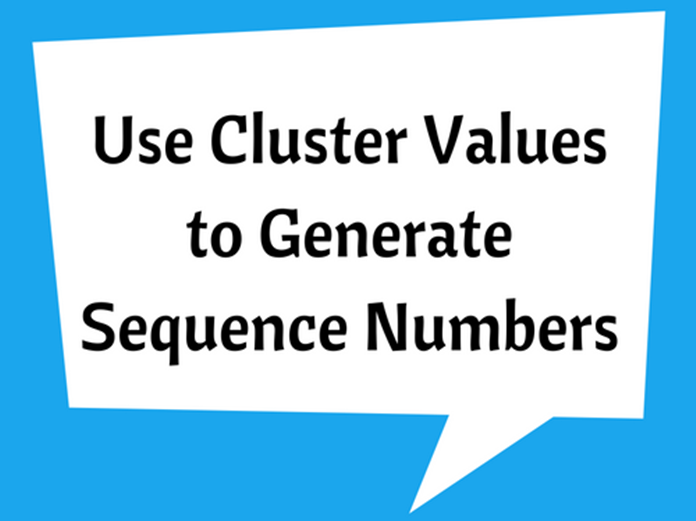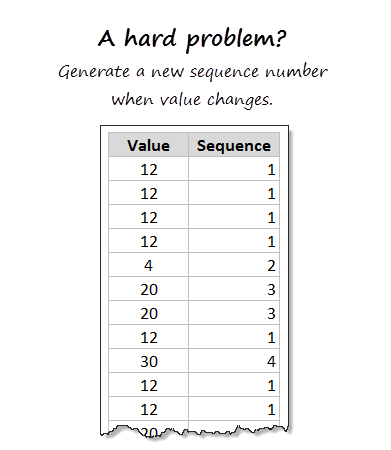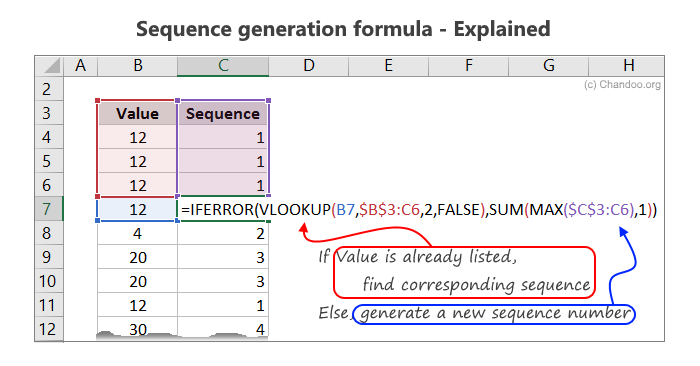# Use Cluster Values to Generate Sequence NumbersIf you are wondering if it is possible to create sequence numbers from clustered values, then you should definitely consider reading ahead to find out more. If a value is already present in the column of data that you are dealing with then you will simply have to obtain the sequence number, if not, you will need to form a new sequence number. You will need to utilize the VLOOKUP formula to conduct this task. For example, assume that the cluster values are located in B4 of Column B and then you will need to write the following formula:

=IFERROR(VLOOKUP(B4,\$B\$3:C3,2,FALSE), SUM(MAX(\$C\$3:C3),1))

It is time for you to understand what the formula is all about.VLOOKUP(B4,\$B\$3:C3,2,FALSE): This portion of the formula will search for the value in the entire column and will try to locate the corresponding values in the next column, i.e. Column C to make the search quick and easy.SUM(MAX(\$C\$3:C3),1)): This portion of the formula will provide you with the next sequence number.

IFERROR(VLOOKUP(…),SUM(…)): This portion of the formula will perform the task of selecting a sequence number or formulating a new number from the provided data.

This formula will help you in arranging the cluster values in order with ease and ranking them in the order that the information has been generated. The formula makes it very quick and simple for you to spot the desired information and create sequence numbers from clustered data making a task that could take hours to complete capable of being done within a matter of minutes. You can now use cluster values to formulate sequence numbers. You can practice generating sequence numbers from cluster values in Excel and learn how to crack this formula. It will take some time getting used to, but eventually you will get there.